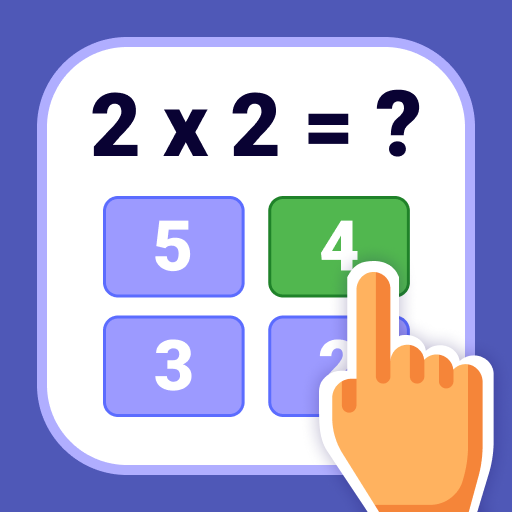👉 Está en:: Mejores apps Android / aplicaciones para aprender inglÃĐs / Multiplication tables - Math learning app

# AnÃĄlisis Multiplication tables - Math learning appMultiplication tables - Math learning app

ðĒ Learn times tables easily by playing! Best multiplication table app ever ðŠ

Learn times tables with one of the coolest Math Games! ðĐâðŦ

We designed multiplication flash cards especially for you. It is essential for students to learn tables 1 to 20 for quick math calculations. So you can practice multiplication and guess all the math puzzles!

Multiplication tables 1 to 30, 40, 50 and even 100 will help you learn and practice the multiplication facts effortlessly. This knowledge is extremely helpful by counting in solving math problems and calculations.

The multiplication app will be useful for both adults and children:
- to master the basics of mathematics and arithmetic, learn the multiplication table, prepare for math tests and exams;
- to keep their mind and brain in good shape.

ð§  Multiplication tables are a practical educational game for everyone.

The multiplications game has three modes: Training mode, Study, Test mode

â Choose the size of the table you want to study (x10 or x20) as well as the type of game - Test, True or False, Input.
â Learn the multiplication table from 1 to 30, and then test your knowledge by solving the multiplication and division examples.
â This Exam Simulator is designed to fix the material. You can choose your own level of complexity (light/middle/complex), and the application will select the intensity according to your level.

After each training you have the opportunity to see which questions have been answered correctly and which have not. This will help next time to improve the result and remember your times tables at home in an easy, step-by-step way.
Start practicing every day, learn to multiply while playing, and get a fast mental calculation. (1x1) ð

Features:

â Simple and intuitive interface
â Cool math learning app for children and adults
â You can train a multiplication table to 10 and 20
â Cards with multiplication table from 1 to 20
â Select the time table you want, study it, review it and become the king of maths
â Intelligent repetition system (look at your errors and try again)
â You will always see the correct answer to each question
â Remember multiplication table in 3 days

Setup the multiplication memorizer and have fun training your brain for school math tests, surveys, exams. Learn times tables playing multiplying games! ðĨ

Math tables are important for basic calculations used during multiplication and division. Your life will be a lot easier when you can simply remember the math multiplication tables! ð

` CategorÃ­a: ` âpara aprender inglÃĐs
` PuntuaciÃģn media:` 4.2529
`Basada en :` 14188 votos
`Desarrollador :` BrainSoft Apps
`Fecha de actualizaciÃģn:` 2021-06-25
`Descarga:` Descargar Multiplication tables - Math learning app